# HBSE 6th Class Maths Solutions Chapter 7 Fractions Ex 7.4

Haryana State Board HBSE 6th Class Maths Solutions Chapter 7 Fractions Ex 7.4 Textbook Exercise Questions and Answers.

## Haryana Board 6th Class Maths Solutions Chapter 7 Fractions Exercise 7.4

Question 1.
Write each fraction. Arrange them in ascending and descending order using correct sign ‘<‘, ‘=’,‘>‘ between the fractions: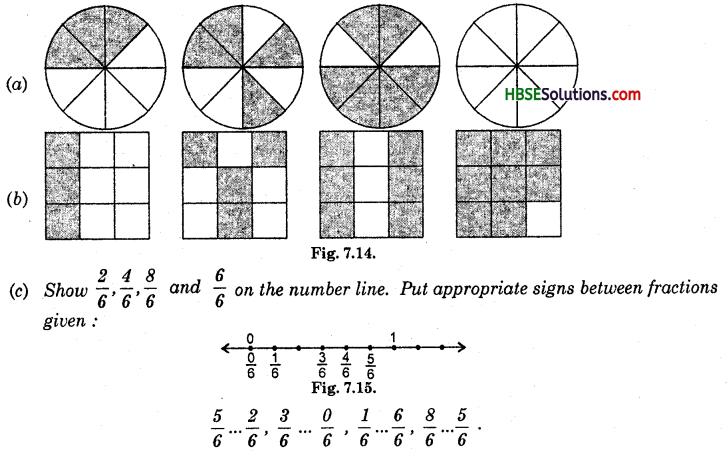Solution: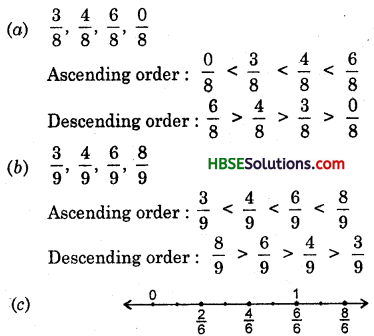Question 2.
Compare the fractions and put an appropriate sign.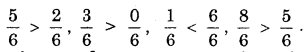Solution: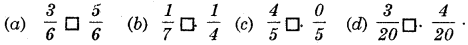Question 3.
Make 5 more such pairs and make appropriate signs.
Solution: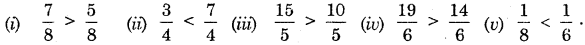Question 4.
Name the fractions and arrange them in ascending order: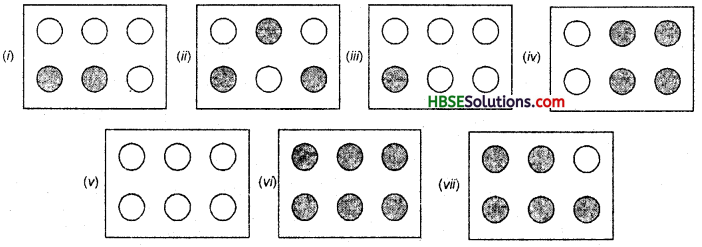Solution: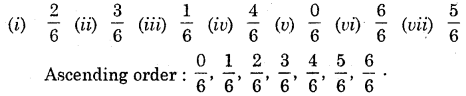Question 5.
Look at the figures and write ‘<‘ or ‘>’, ‘=’ between the pairs of fractions.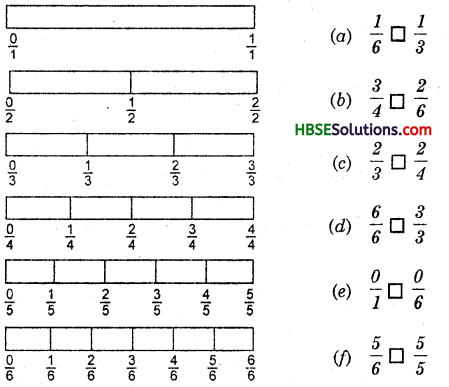Make 5 more such problems and solve them with your friends.
Solution: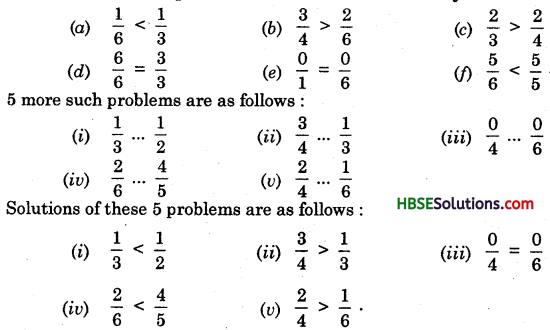Question 6.
How quickly can you compare these? Fill appropriate signs.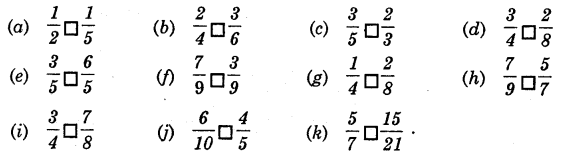Solution: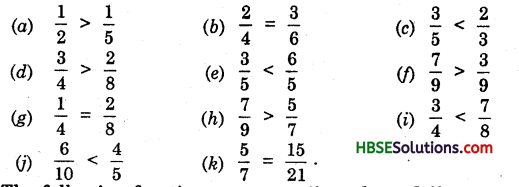Question 7.
The following fractions represent just three different numbers. Separate them into three grups of equal fractions by changing each one to its simplest fraction.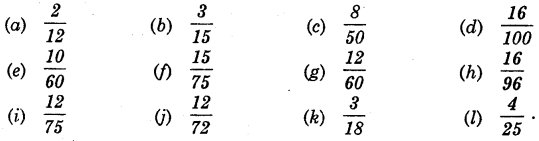Solution: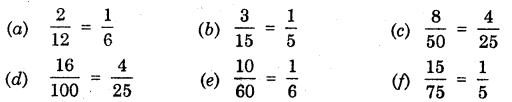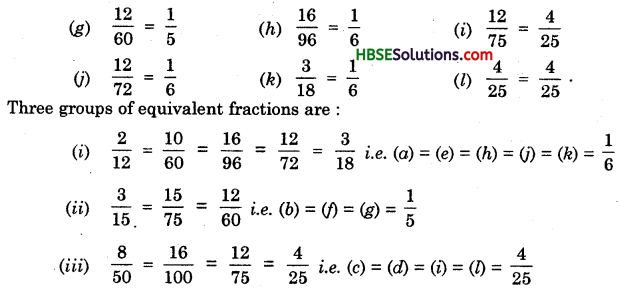Question 8.
Find answers to the following. Write and indicate how you solved them:
(a) Is $$\frac{5}{9}$$ equal to$$\frac{4}{5}$$ ?
(b) Is $$\frac{9}{16}$$ equal to $$\frac{5}{9}$$ ?
(e) Is $$\frac{4}{5}$$ equal to $$\frac{16}{20}$$?
(d) Is $$\frac{1}{15}$$ equal to $$\frac{4}{30}$$?
Solution:
(a) $$\frac{5}{9} \neq \frac{4}{5}$$ because 5 x 5 ≠ 9 x 4 i.e., 25 ≠ 36
(b) $$\frac{9}{16} \neq \frac{5}{9}$$ because 9 x 9 ≠ 16 x 5 i.e., 81 ≠ 80
(c) $$\frac{4}{5}=\frac{16}{20}$$ because 4 x 20 = 5 x 16 i.e., 80 = 80
(d) $$\frac{1}{15} \neq \frac{4}{30}$$ because 1 x 30 ≠ 15 x 4 i.e., 30 ≠ 60.Question 9.
Ila read 25 pages of a book containing 100 pages. Lalita read $$\frac{1}{2}$$ of the same book. Who read less ?
Solution:
Pages read by Ila = 25
Pages read by Lalita = $$\frac{1}{2}$$ x 100 = 50
∵ 25 < 50

Question 10.
Rafiq exercised for $$\frac{3}{6}$$ of an hour, while Rohit exercised for $$\frac{3}{4}$$ of an hour.
Who exercised for a longer time ?
Solution:
Rafiq exercised for $$\frac{3}{6}=\frac{1}{2}$$ of an hour = $$\frac{1}{2}$$ x 60 = 30 min.
Rohit exercised for $$\frac{3}{4}$$ of an hour = $$\frac{3}{4}$$ x 60 = 45 min.
∴ Rohit exercised for a longer time.Question 11.
In a class A of 25 students 20 passed in first class; in another class B of 30 students, 24 passd in first class. In which class were there more number of students getting first class ?
Solution:
Fraction of the students passed in first class in class A = $$\frac{20}{25}=\frac{4}{5}$$
Fraction of the students passed in first class in class B = $$\frac{24}{30}=\frac{4}{5}$$
∴ Equal number of students got first class in both the classes.